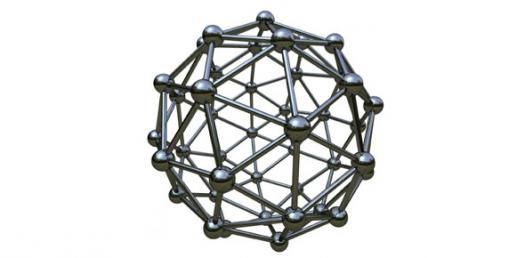# Average Atomic Masses Quiz

8 Questions | Total Attempts: 148SettingsRelated Topics
• 1.
Calculate the relative formula mass for the compound: H2SO4
• A.

96

• B.

97

• C.

98

• D.

99

• 2.
Calculate the relative formula mass for the compound: Fe2(SO4)3
• A.

399

• B.

400

• C.

401

• D.

402

• 3.
Calculate the relative formula mass for the compound: NAOH
• A.

39

• B.

40

• C.

41

• D.

42

• 4.
Calculate the relative formula mass for the compound: HNO3
• A.

63

• B.

64

• C.

65

• D.

66

• 5.
Calculate the relative formula mass for the compound: FeO
• A.

69

• B.

70

• C.

71

• D.

72

• 6.
Calculate the relative formula mass for the compound: CaCl
• A.

109

• B.

110

• C.

111

• D.

112

• 7.
Calculate the relative formula mass for the compound: C2H5OH
• A.

46

• B.

47

• C.

48

• D.

49

• 8.
Calculate the relative formula mass for the compound: (NH4)2SO4
• A.

131

• B.

132

• C.

133

• D.

134